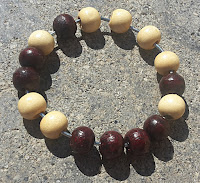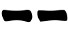## Saturday 18 June 2016Another method taken from Russell Cottrell site: memory wheels.
They are sequences of black and white beads (representing yin and yang lines) that encode the entire set of sixtyfour hexagrams. The picture below, from Russel's site, shows one of those wheels:

Start fom any bead, consider it with the next five beads and draw the hexagram; then move to the next bead and do the same.  After having taken all the 64 beads, you will have drawn all the possible 64 hexagram with no repetition!

This seemingly magic property is due to the fact that the beads are arranged in a so called De Bruijn sequence: a way to represent a sequence of all the numbers between 0 and 2n-1 using just 2n bits instead of  n*2n  bits. They have many uses in the field of probability theory, coding and telecommunication.

The compression property they have, makes them a good tool to aid memorization of long sequences of numbers, hence the name of memory wheels. They are reported to be in use since ancient times.

There are many De Bruijn sequence for the sixtyfour I Ching hexagrams; to be precise there are  226 = 67 108 864 of such sequences, each one encoding a different sequence of hexagrams. The following are just two example (0 stands for Black, 1 for white) taken from Russell's site:

0000001111010000100010100100110001101010110010111001110110111111
0000001111010011101111110011011010111000110010110000101010001001

you can find many more there.

De Bruijn sequences have been used to create discs, bracelets, wheels and necklaces related to the I Ching, not all of them, however, for the purpose of divination. There is an interesting summary in the Forum hosted on Clarity on Line.

A wheel like the one in the picture above has no begininng and no end, it is perfect for casting hexagrams as follows:
1. Select, without looking, a bead from the wheel;
2. Draw the line based on the color of the bead:
• If it's black, draw;
• If it's white, draw;
3. Pick the next bead in the sequence (the direction is not important as long as you are consistent);
4. Repeat steps 2-3 five more times to get the hexagram.
To get moving lines Russell proposes to use the wheel to draw the hexagram one line at the time and I will describe them in next section.

On Clarity, charly suggested to directly generate the secondary hexagram exactly as done for the primary and determine the moving lines by comparing the two. This approach, which can be used with any method that casts a hexagram at once like the I Ching decks, would provide moving lines with probabilities  1/4.

A more portable device would use less beads. I've built one with sixteen beads as shown in the picture below.

### Probabilites

We can use both the 64 wheel designed by Russell or the 16 wheel shown above, to generate lines with different probability distributions using the following methods.

#### Three coins probabilities

1. Select, without looking, a bead from the wheel;
2. Draw the line based on the color of the bead:
• If it's black, draw;
• If it's white, draw;
3. Look at next two beads in the sequence, if all the three beads are of the same, it's a moving line;
4. Repeat steps 1-3 five more times drawing the hexagram from bottom to top;
A De Bruijn sequence with sixteen beads encodes a sequence of 16 numbers between 0 and 8 where each number appears exactly twice. Requiring that moving lines are generated by a sequence of three beads of the same color means we have the following possibilities (B for Black, W for white):

BBB 6    BBW 8    BWB 8    BWW 8
WWW 9    WBW 7    WWB 7    WBB 8

From which we can see that:
Prob(6) = Prob(9) = 1/8 = 12.5%
Prob(8) = Prob(7) = 3/8 = 37.5%
Prob(yin) = Prob(yang) = 1/2
like in the three coins method.

Note that if you just want three coins probabilities and want something smaller, you can build a wheel using eight beads with the following sequence:   B B B W B W W W

#### Yarrow stalks probabilities

1. Select, without looking, a bead from the wheel;
2. Draw the line based on the color of the bead:
• If it's black, draw;
• If it's white, draw;
3. Look at the next three beads in the sequence, if you can see exactly three white beads among the four beads, it's a moving line;
4. Repeat steps 1-3 five more times drawing the hexagram from bottom to top;
This is equivalent to the four coins method with white as head and black as tail.

#### Equal probabilities

1. Select, without looking, a bead from the wheel;
2. Draw the line based on the color of the bead:
• If it's black, draw;
• If it's white, draw;
3. If the next bead in the sequence is of the same color of the selected one, it's a moving line;
4. Repeat steps 1-3 five more times drawing the hexagram from bottom to top;
Reasoning as we did for the three coins probabilities above, we have (B for Black, W for white):
BB 6    BW 8
WW 9    WB 7

From which we get:
Prob(6) = 1/4 = 25%
Prob(8) = 1/4 = 25%
Prob(7) = 1/4 = 25%
Prob(9) = 1/4 = 25%
Prob(yin) = Prob(yang) = 1/2

Note that if you just want equal probabilities and want something smaller, you can build a wheel using four beads with the following sequence:   B B W W